# Explanation of results

## Current crossing a surface

If the surface of the S area is perpendicular to the current lines and the current density has the same value J at any point on this surface, the electric current crossing the surface is: I = J S

For a surface whose normal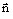is randomly directed with respect to the current density vector, then the electric current crossing the surface is: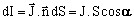The current flowing within an undetermined surface will be: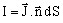## Dissipated power / losses by Joule effect

The density of the dissipated power (or density of losses by Joule effect) at a point is expressed by means of E and J by the relation: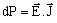that can also be written as:

dP = ρ J2 = σE2

The dissipated power (or losses by Joule effect) on a volume region is equal to: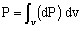## Electric resistance of a conductor

The electric current flowing through a portion of a conductor (i element) can be expressed by the relation: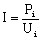where:

• Ui is the voltage at the terminals of the conductor element i
• Pi are the losses by Joule effect in the conductor element i

The electric resistance of a portion of a conductor (element i) can be written as: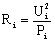## Laplace's force

The force exerted on a non-magnetic conducting region can be calculated by the Laplace's law: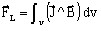Attention: It is necessary to calculate first the magnetic flux density BJ